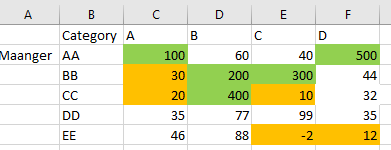# QlikView App Dev

Discussion Board for collaboration related to QlikView App Development.

Announcements
Read about the latest Qlik Community enhancements on the Community News blog!
cancel
Showing results for
Did you mean:Creator

## Rank in Pivot table

Hi, We have a requirement to highlight the top 5 and bottom 5 values in the pivot table. We can use the rank function to get the top 5 but I am unable to understand how to write this function for the cross table format of the pivot table.

I have attached the screenshot of the sample data with the expected output.I searched before posting but did not get the info.

1 Solution

Accepted SolutionsMVP

You might have to use Aggr() function here

``Aggr(If(Rank(TOTAL Sum(Measure)) < 6, Green(), If(Rank(TOTAL -Sum(Measure)) < 6, Yellow())), Maanger, Category)``
6 RepliesMVP

Do you have any other dimension in pivot table?  If so how would you want to show Top5?Creator
Author

I don't have any other dimension, I just need to highlight the top5 and bottom 5 values.

in the attachment, i have highlighted the top 5 in green and bottom 5 in the amber color the same way I want to show.MVP

You might have to use Aggr() function here

``Aggr(If(Rank(TOTAL Sum(Measure)) < 6, Green(), If(Rank(TOTAL -Sum(Measure)) < 6, Yellow())), Maanger, Category)``MVP

Assuming you have two dimensions of which one is dragged to column. You can write the below expression in background color

Assuming you have Sum(Value) as your expression

=if(sum(Value)>= max(total aggr(sum(Value),Category,Name),4),green(),
if(sum(Value)<= min(total aggr(sum(Value),Category,Name),4),red()))MVP

@sunny_talwar  gotcha!!!Creator
Author

Thanks SunnyCommunity Browser